# Carathéodory conditions

Jump to: navigation, search

If one wants to relax the continuity assumption on a functionwhile preserving the natural equivalence between the Cauchy problem for the differential equationand the integral equation which can be obtained by integrating the Cauchy problem, one can follow ideas of C. Carathéodory [a1] and make the following definition.

Letbe an open set and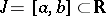,. One says that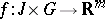satisfies the Carathéodory conditions on, written as, if

1)is measurable for every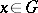(cf. also Measurable function);

2)is continuous for almost every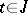;

3) for each compact setthe function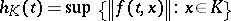is Lebesgue integrable (cf. also Lebesgue integral) on, where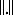is the norm in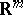.

If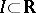is a non-compact interval, one says thatsatisfies the local Carathéodory conditions on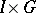if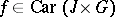for every compact interval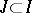. This is written as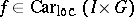.

Note that any functionwhich is the composition ofand a measurable function, i.e.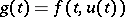(cf. also Composite function), is measurable on.

To specify the space of the majorantmore precisely, one says thatis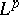-Carathéodory,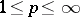, ifsatisfies 1)–3) above with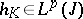.

One can see that any function continuous on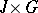is-Carathéodory for any.

Similarly, one says thatis locally-Carathéodory onifrestricted tois-Carathéodory for every compact interval.